## Consider the following results from the last ten periods of student enrollment forecast by the Operations Management department chairman. Pe

Question

Consider the following results from the last ten periods of student enrollment forecast by the Operations Management department chairman. Period Forecast Actual 1 25 26 2 32 31 3 42 45 4 53 50 5 64 70 6 70 72 7 81 78 8 88 90 9 95 100 10 102 110 Determine the mean absolute percentage error (MAPE, round to two decimal places). Enter answer as decimal. (10% should be entered as .1)

in progress 0
5 months 2021-08-03T00:49:19+00:00 1 Answers 22 views 0

## Answers ( )

MAPE = 2.33%

Step-by-step explanation:

The mean absolute percentage error (MAPE) can be determined by:

MAPE =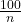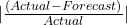Given that:

periods are 1, 2, 3, 4, 5, 6, 7, 8, 9, 10

forecast are 25, 32, 42, 53, 64, 70, 81, 88, 95, 102

actual are 26, 31, 45, 50, 70, 72, 78, 90, 100, 110

Thus, n = 10 and;= |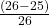+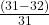+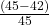+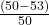+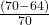+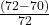+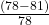+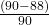+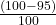+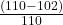|

= |0.0385 + (-0.0323) + 0.0667 + (-0.06) + 0.0857 + 0.0278 + (-0.0385) + 0.0222 + 0.05 + 0.07273|

= 0.23283

Therefore;

MAPE =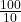x 0.23283

= 10 x 0.23283

= 2.3283%

= 2.33%

The mean absolute percentage error = 2.33%